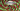# Rotate Array - Leet Code Solution

August 28, 2020## Problem Statement

Given an array, rotate the array to the right by k steps, where k is non-negative.

Example

``````Input: nums = [1,2,3,4,5,6,7], k = 3
Output: [5,6,7,1,2,3,4]``````

## Solution (Simple)

Its like shifting array to right one by one. Lets us assume if you were to shift array by one, what will you do.

``````1,2,3,4,5,6,7

# Shift one time
7,1,2,3,4,5,6``````

You could take backup of the last element of array, and start shifting previous element in next array location one by one. And if you have to do this for `k` shifts, you could have a counter loop for that.

### Code

``````public void rotate(int[] nums, int k) {
if (k==0) return;
if (nums == null || nums.length == 0) return;

for (int i=0; i<k; i++) {
int j=nums.length-1;
int temp = nums[j];
for (; j>0; j--) {
nums[j] = nums[j-1];
}
nums = temp;
}
}``````

### Explanation

• First loop is for the counter, how many times we need to rotate (shift)
• For inner loop, we are starting from the end of array.
• Take copy of last element. Start copying previous element in next array location.
• In last, put last copied element to first location.

### Complexity

The code runs in `O(k*n)`

## Better Solution with Extra Space

If you look closely the input and expected output. You will realize that we don’t need to shift array one by one. We can directly put an array element to its respective position.

``````# Input
1,2,3,4,5,6,7

k=3

#Output
[5,6,7,1,2,3,4]``````

Example for `index-0`, we need to copy it to `index-0 + k` index. For later index, this value might exceeds the length of array. For that, we can take a mod of length.

``````#for a current index=currentIndex
targetIndex = (currentIndex + k) % length``````

Lets do this in an extra array

### Code

``````public void rotate_extraspace(int[] nums, int k) {
if (k==0) return;
if (nums == null || nums.length == 0) return;

int[] res = new int[nums.length];
for (int i=0; i<nums.length; i++) {
int newIndex = (i + k) % nums.length;
res[newIndex] = nums[i];
}

//assign back to original array
for (int i=0; i<nums.length; i++) {
nums[i] = res[i];
}
}``````

### Complexity

• Time Complexity `O(n)` We are iterating over array two times.
• Space Complexity `O(n)`

## Best Solution with No Extra Space

We want a solution with no extra space. It should be clear that above method works well, but we need to have extra space to take backups of overwritten indexes.

We can definitely think of taking one or two variables to take such backup. And, iterate over array.

### Understanding the Algorithm

``````# Input
[1,2,3,4,5,6,7]

# Solution
## startIndex=0, k=3
## targetIndex=(startIndex + k) % length = 3

## Copy index-0 value to index-3, but we need to take backup of index-3 as we have to copy this value to its target index as well.``````

So, if we keep iterating like this starting from an index. We will cover all such jumps with current index. And, finally we will reach to the same index after such iteration.

Lets see with example:

``````[1,2,3,4,5,6,7]

#startIndex=0, k=3

## Output after iteration-1
## [1,2,3,1,5,6,7], backup=4, targetIndex=3

## Output after iteration-2
## [1,2,3,1,5,6,4], backup=7, targetIndex=6

## Output after iteration-3
## [1,2,7,1,5,6,4], backup=3, targetIndex=2

## Output after iteration-4
## [1,2,7,1,5,3,4], backup=6, targetIndex=5

## Output after iteration-5
## [1,6,7,1,5,3,4], backup=2, targetIndex=1

## Output after iteration-6
## [1,6,7,1,2,3,4], backup=5, targetIndex=0

# reached to same index from where we started, index-0
## Copy backup element to targetIndex
## [5,6,7,1,2,3,4]``````

The above example compled when we started with index-0. But, it will not work with below example:

``-1,-100,3,99, k=2``

What we need is another loop over our above logic, which will just increment our current index to next, and we will repeat our logic again.

Lets see:

### Code with Test cases

``````package com.gyanblog.leetcode;
import com.gyanblog.utils.ArrayUtils;

public class Q189_RotateArray {
public void rotate(int[] nums, int k) {
if (k==0) return;
if (nums == null || nums.length == 0) return;

int count = 0;
for (int i=0; i<nums.length && count < nums.length; i++) {

int currentIndex = i;
int newIndex = (currentIndex + k) % nums.length;

int currentTemp = nums[currentIndex];
int nextTemp;

while (newIndex != i) {
nextTemp = nums[newIndex];
nums[newIndex] = currentTemp;
currentIndex = newIndex;

newIndex = (currentIndex + k) % nums.length;
currentTemp = nextTemp;

//how many replacements has been done
count ++;
}
//assign value from where we started
nums[newIndex] = currentTemp;
count ++;
}
}

public static void main(String[] args) {
System.out.println("Test-1 status=" + (test1() ? "pass" : "fail"));
System.out.println("Test-2 status=" + (test2() ? "pass" : "fail"));
}

public static boolean test1() {
Q189_RotateArray q = new Q189_RotateArray();
int[] nums = {1,2,3,4,5,6,7};
int k = 3;

q.rotate(nums, k);
return ArrayUtils.compareArray(nums, new int[] {5,6,7,1,2,3,4}, nums.length);
}

public static boolean test2() {
Q189_RotateArray q = new Q189_RotateArray();
int[] nums = {-1,-100,3,99};
int k = 2;

q.rotate(nums, k);
return ArrayUtils.compareArray(nums, new int[] {3,99,-1,-100}, nums.length);
}
}``````

### Complexity

Its `O(n)` You might be wondering, since we are running two loops. If you see, we are iterating only till the length of the array.

You can see the github code for this problem